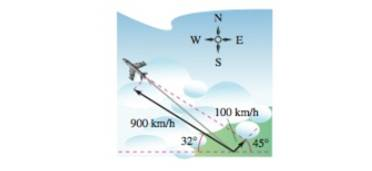Chapter 11.1, Problem 83E

Chapter
Section
Textbook Problem

# Navigation A plane is flying with a bearing of 302 ∘ . Its speed with respect to the air is 900 kilometers per hour. The wind at the plane舗s altitude is from the southwest at100 kilometers per hour (see figure). What is the true direction of die plane, and what is its speed with respect to the ground?To determine
The true direction of the plane and speed with respect to ground.

Explanation

Given: Speed of wind 100 km/hr from south west speed of plane 900km/hr and bearing of 302°ï¿½

Explanation: Angle of plane with positive x-axis is -58°ï¿½ Angle of wind with positive x-axis is 45°ï¿½

velocity component of plane (v1) = 900cos(58),sin(58)                                                  =476.927i763.243jvelocity component of plane (v2) = 100cos45,sin45                                                  =502i+502jTrue direction of plane (v)=v1+v2=476

### Still sussing out bartleby?

Check out a sample textbook solution.

See a sample solution

#### The Solution to Your Study Problems

Bartleby provides explanations to thousands of textbook problems written by our experts, many with advanced degrees!

Get Started